## 6.1 Solutions for assessment of mobile network data energy efficiency

28.3103GPPEnergy efficiency of 5GManagement and orchestrationRelease 18TS

### 6.1.1 Energy efficiency of NG-RAN

Assessment of NG-RAN data EE is based on the high-level mobile network data EE KPI defined in clause 3.1 and clause 5.3 of ETSI ES 203 228 :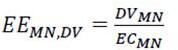For different gNB scenarios, the two following performance measurements may be used as the DVMN:

– For split-gNBs scenario:

1) DL PDCP SDU Data Volume per interface (cf. clause 5.1.3.6.2.3 of TS 28.552 ): This measurement provides the Data Volume (amount of PDCP SDU bits) in the downlink delivered from GNB-CU-UP to GNB-DU (F1-U interface), to external gNB-CU-UP (Xn-U interface) and to external eNB (X2-U interface). The measurement is calculated per QoS level (mapped 5QI or QCI in NR option 3) and per S-NSSAI and per PLMN ID, and reported per Interface (F1-U, Xn-U, X2-U);

2) UL PDCP SDU Data Volume per interface (cf. clause 5.1.3.6.2.4 of TS 28.552 ): This measurement provides the Data Volume (amount of PDCP SDU bits) in the uplink delivered to GNB-CU-UP from GNB-DU (F1-U interface), from external gNB-CU-UP (Xn-U interface) and from external eNB (X2-U interface). The measurement is calculated per QoS level (mapped 5QI or QCI in NR option 3) and per S-NSSAI and per PLMN ID, and reported per Interface (F1-U, Xn-U, X2-U);

– For non-split gNBs scenario:

1) DL Cell PDCP SDU Data Volume (cf. clause 5.1.2.1.1.1 of TS 28.552 ): This measurement provides the Data Volume (amount of PDCP SDU bits) in the downlink delivered to PDCP layer. The measurement is calculated per PLMN ID and per QoS level (mapped 5QI) and per S-NSSAI;

2) UL Cell PDCP SDU Data Volume (cf. clause 5.1.2.1.2.1 of TS 28.552 ): This measurement provides the Data Volume (amount of PDCP SDU bits) in the uplink delivered from PDCP layer to higher layers. The measurement is calculated per PLMN ID and per QoS level (mapped 5QI) and per S-NSSAI;

The following PEE (Power, Energy and Environmental) measurement may be used as the ECMN:

– PNF Energy consumption (cf. clause 5.1.1.19.3 of TS 28.552 ): This measurement provides the energy consumed (in kilowatt-hours) by the subject gNB.

### 6.1.2 Energy efficiency of network slices

#### 6.1.2.1 Introduction

Assessment of the energy efficiency of 5G network slices is based on KPIs defined in TS 28.554  clause 6.7.2.

#### 6.1.2.2 Generic Network Slice Energy Efficiency (EE) KPI

The Generic network slice EE KPI is defined as: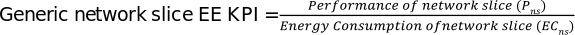, where ‘Performance of network slice’ (Pns) is defined per type of network slice.

#### 6.1.2.3 Energy efficiency of eMBB network slice

This KPI is obtained by the sum of UL and DL data volumes at N3 interface(s) of the network slice, divided by the energy consumption of the network slice. The unit of this KPI is bit/J.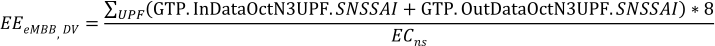The following measurements are used:

– GTP.InDataOctN3UPF.SNSSAI: Number of octets of incoming GTP data packets on the N3 interface, from (R)AN to UPF, where SNSSAI identifies the S-NSSAI, as defined in TS 28.552  clause 5.4.1.3;

– GTP.OutDataOctN3UPF.SNSSAI: Number of octets of outgoing GTP data packets on the N3 interface, from (R)AN to UPF, where SNSSAI identifies the S-NSSAI, as defined in TS 28.552  clause 5.4.1.4.

#### 6.1.2.4 Energy efficiency of URLLC network slice

##### 6.1.2.4.1 Introduction

This KPI is defined with two variants.

##### 6.1.2.4.2 Based on latency of the network slice

This KPI is obtained by the inverse of the average end-to-end User Plane (UP) latency of the network slice divided by the energy consumption of the network slice. The unit of this KPI is (0.1ms * J)-1.

In this KPI variant, latency is the only factor considered for evaluating the performance of network slice, i.e. the performance of network slice (Pns) for URLLC type of network slice is the inverse of the end-to-end User Plane (UP) latency of the network slice.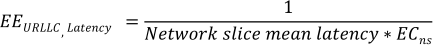Where Network slice mean latency is defined as: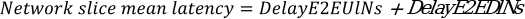The following KPIs are used to calculate Network slice mean latency:

– DelayE2EUlNs: Average e2e uplink delay for a network slice, defined in TS 28.554  clause 6.3.1.8.1 as the average e2e UL packet delay between the PSA UPF and the UE for a network slice;

– DelayE2EDlNs: Average e2e downlink delay for a network slice, defined in TS 28.554  clause 6.3.1.8.2 as the average e2e DL packet delay between the PSA UPF and the UE for a network slice.

##### 6.1.2.4.3 Based on both latency and Data Volume (DV) of the network slice

This KPI is obtained by the product of the sum of UL and DL traffic data volumes at N3 interface(s) of the network slice multiplied by the inverse of the end-to-end User Plane (UP) latency of the network slice, divided by the energy consumption of the network slice. The unit of this KPI is bit/(0.1ms*J).

In this KPI variant, data volume and latency are two factors considered for evaluating the performance of network slice, i.e. the performance of network slice (Pns) for URLLC type of network slice is the sum of UL and DL traffic data volumes at N3 interface(s) multiplied by the inverse of the end-to-end User Plane (UP) latency of the network slice. This solution is applicable for the case of URLLC network slice is deployed and operators want to evaluate the slice EE KPI for different periods of time such as busy time slots (more DV) and idle time slots (less DV).The following measurements, defined in TS 28.552 , are used to calculate the sum of UL and DL traffic data volumes at N3 interface(s) of the network slice:

– GTP.InDataOctN3UPF.SNSSAI: Number of octets of incoming GTP data packets on the N3 interface, from (R)AN to UPF), where SNSSAI identifies the S-NSSAI, as defined in TS 28.552  clause 5.4.1.3;

– GTP.OutDataOctN3UPF.SNSSAI: Number of octets of outgoing GTP data packets on the N3 interface, from (R)AN to UPF), where SNSSAI identifies the S-NSSAI, as defined in TS 28.552  clause 5.4.1.4.

The following KPIs, defined in TS 28.554 , are used to calculate the latency of the network slice:

– DelayE2EUlNs: Average e2e uplink delay for a network slice, defined in TS 28.554  clause 6.3.1.8.1 as the average e2e UL packet delay between the PSA UPF and the UE for a network slice;

– DelayE2EDlNs: Average e2e downlink delay for a network slice, defined in TS 28.554  clause 6.3.1.8.2 as the average e2e DL packet delay between the PSA UPF and the UE for a network slice.

#### 6.1.2.5 Energy efficiency of MIoT network slice

##### 6.1.2.5.1 Introduction

This KPI is defined with two variants.

##### 6.1.2.5.2 Based on the number of registered subscribers of the network slice

This KPI is obtained by the mean number of active UEs of the network slice divided by the energy consumption of the network slice. The unit of this KPI is UE/J.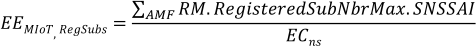The following measurement is used:

– RM.RegisteredSubNbrMax.SNSSAI: maximum number of registered state subscribers per AMF, where SNSSAI identifies the S-NSSAI, as defined in TS 28.552  clause 5.2.1.2.

##### 6.1.2.5.3 Based on the number of active UEs in the network slice

This KPI is obtained by the mean number of active UEs of the network slice divided by the energy consumption of the network slice. The unit of this KPI is UE/J.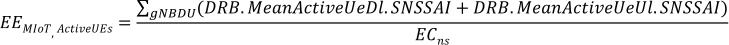The following measurements are used:

– DRB.MeanActiveUeDl.SNSSAI, where SNSSAI identifies the S-NSSAI, as defined in TS 28.552  clause 5.1.1.23.1.

– DRB.MeanActiveUeUl.SNSSAI, where SNSSAI identifies the S-NSSAI, as defined in TS 28.552  clause 5.1.1.23.3.